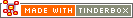^max( group , attribute[, precision] )^Code Type: Calculation Code Scope of Action: group

^max( group , attribute[, precision] )^

Returns the attribute value for the note within the group whose attribute value is the largest.

An optional third argument lets you set the number of decimal points that are exported by the numeric group mark-up elements(^sum^, ^min^, ^max^, ^mean^, ^count^). Note that the precision is always the third argument.

For internal use, see the max() operator.

 Up: Group-based Previous: ^every( group , attribute[, data] )^ Next: ^mean( group , attribute[, precision] )^

[Last updated: 14 Dec 2009, using v5.0]